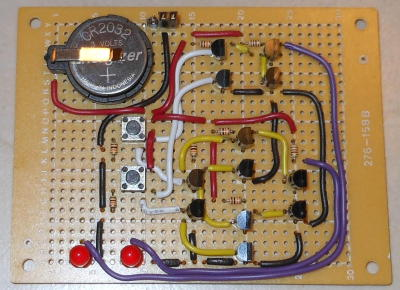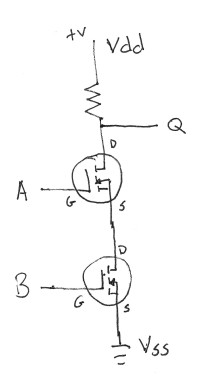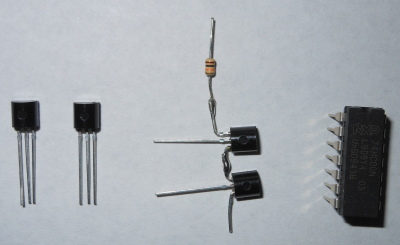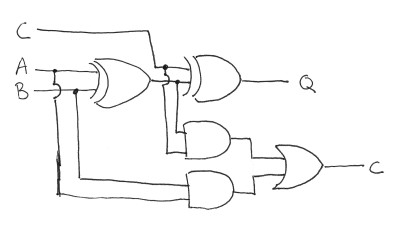CONTENTS

Home
Software
Electronics
Music
Resume
Contact

GitHub

Posted: December 16, 2015

Introduction

Here's a small piece of electronic artwork: a half adder made out of transistors. This is really a pretty basic circuit, although as seen from the wires on the circuit below, kind of a pain in the neck to wire up. This project ends up being an extension of an earlier XOR gate project I did with the difference being it wasn't done with conductive ink and there is also an AND gate added.

The circuit, if left on for a little while, will start to fade on the LEDs (seems mostly the carry bit here). I kind of assumed it was because I left out 10k pull-down resistors between the gate and base of the 2N7000 MOSFETs. It seems it's because I didn't notice the gate threshold is 3.2v on the 2N7000, for some reason I thought it was below 3v. The coin battery is 3v, which isn't enough to drive the MOSFET fully open. When I ran it at 4.5v it behaves.

Below is the half adder circuit made from 2N7000 MOSFETs. The two LED's at the bottom left represent the answer to the math problem button 1 + button 2. The buttons are lined up above the 1's column and the LED on the left is the 2's column (can also be thought of as the carry bit).So basically the a half adder is simply an XOR gate (the cluster of 8 transistors in a diamond formation at the lower part of the circuit) to calculate the value of addition between two 1 bit binary numbers. The carry bit is only set if both digits in the addition are 1's, so this is just simply an AND gate (the cluster of 4 transistors above the XOR gate).Since all logic gates (NAND, AND, NOR, OR, XOR, NOT) can simply be made out of NAND gates, the adder can be broken up into a set of NAND gates:And since NAND gates are simply made out of two transistors, the adder can be broken down one more time into a set of transistors. In the circuit above I made each NAND gate out of 2 MOSFETs in the configuration below.NAND gates could also be made from bipolar NPN transistors:Below, from left to right, two 2N7000 MOSFET transistors, two 2N7000's soldered together to make a NAND gate, and a 7400 IC quad NAND gate IC.To make the circuit into a full adder, a carry bit would be included into the equation. This would require 2 XOR gates for bit 0 and to calculate carry, the equation (A AND B) OR ((A XOR B) AND C) would be used.Related Projects @mikekohn.net